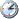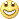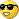# Raw Ideas - page 13[Deleted]315

New system Based on Fibonacci

Here is a system I found in mt3 and converted it to mt4.

It looks like it may have some promise. but currently it has 1 flaw that I see.

The lines do not refreash, so you need to delete the indicator each day then reinstall it.

I believe the indicator just needs a delete object code installed, but I have not had the time, perhaps later in the month. as I said it works fine if you remove it from the chart, then reinstall (start of each session).

I should aslo add that it uses the zig-zag indicator, si you also need it in the indicators directory

Enjoy

Files:121

trending system?

I am comtemplating building a automatic trading system based on C. Youngs APE indicator. The part of the indicator I want to base it on is the "Alpha" indicator. In short the alpha indicator is very similar to the ADX or range action verification index, see stocks commodities mag april 2006. This alpha indicator is more responsive then the traditional adx etc.. It goes into a trend much quicker and more importantly it comes out of a trend quicker.1177

SnapshotI

Hello,

I found this indicator which will enable you to make scrrenshoot of your diagram.

This means that it can be attached to any chart where you want a image

To put in the Folder of the indicators and the images is in the folder :Files

Files:173

Coding Modification for EA needed

The following EA is based on the Parabolic Sar. It works very well but what I am struggling with is the following. The EA is based on a 30min EUR/USD chart. In a fast moving market the EA will open and close 2 or more trades on the same bar. I want it to only trade once on a 30min bar. Once a trade has been executed and closed it MUST NOT trade for the next 30minutes. Any help greatly appreciated. Many thanks

Another thing that would really improve this EA. When the first dot appears it enters almost at the end of the bar (end of 30mins). If possible I would like it to enter the trade as soon as the first dot appears above or below the bar.

Best regards

Files:
par5.mq4 9 kb16

anyone seen a candle or bar range indictor?

Hey all, Anyone know of a candle or bar indicator which can be set to alert if a certain range is hit during that candle or bar. Example a doji with zero pip range between open and close price or on the other extreme fifty pip range in the candle or bar, and when the bar or candle closes if the set number is hit an alert? Thanks Rip75

Order Pending

Hi,

Code would like it so that the EA places only 3 orders SELLLIMIT or BUYLIMIT of each time, keeping these orders for 3 hours. Or either necessary of a limitor of hanging orders, my system has placed orders of uninterrupted form and 3 are only necessary.

Grateful285

Fuzzy Expert System Example

HelloJust go to the following page :

http://merlotti.com/EngHome/Computing/software.htm

You'll find Docs, Example and Source Code ( modifiable ).....

Good exploration.....
DV75

Help me please - Order Pending

Hi,

Code would like it so that the EA places only 3 orders SELLLIMIT or BUYLIMIT of each time, keeping these orders for 3 hours. Or either necessary of a limitor of hanging orders, my system has placed orders of uninterrupted form and 3 are only necessary.

Grateful

Total=OrdersTotal();

if(OrdersTotal()==0)

{b1=0;b2=0;b3=0;s1=0;s2=0;s3=0;}

if(OrdersTotal()>0)

{

//Print("Total Orders:",OrdersTotal());

//Print(b1," ",b2," ",b3," ",s1," ",s2," ",s3);

for(cnt=0;cnt<Total;cnt++)

{

if(OrderMagicNumber()==2)

{b1=OrderTicket(); }

if(OrderMagicNumber()==4)

{b2=OrderTicket(); }

if(OrderMagicNumber()==6)

{b3=OrderTicket(); }

if(OrderMagicNumber()==1)

{s1=OrderTicket(); }

if(OrderMagicNumber()==3)

{s2=OrderTicket(); }

if(OrderMagicNumber()==5)

{s3=OrderTicket(); }

}

}

double expirar=(CurTime()+PERIOD_H1*120);

if(b1==0)

{

if (Order == SIGNAL_BUY && ((EachTickMode && !TickCheck) || (!EachTickMode && (Bars != BarCount)))) {

//Check free margin

if (AccountFreeMargin() < (1000 * Lots)) {

Print("We have no money. Free Margin = ", AccountFreeMargin());

return(0);

}

if (StopLossMode) StopLossLevel = Ask - StopLoss * Point; else StopLossLevel = 0.0;

if (TakeProfitMode) TakeProfitLevel = Ask + TakeProfit * Point; else TakeProfitLevel = 0.0;

if(Ticket > 0)

{

if (OrderSelect(Ticket, SELECT_BY_TICKET, MODE_TRADES)) Print("BUY order opened : ", OrderOpenPrice()); else Print("Error opening BUY order : ", GetLastError());

{

b1=Ticket;

Print(Ticket);}

if (EachTickMode) TickCheck = True;

if (!EachTickMode) BarCount = Bars;

return(0);

}

}

}

}

if (b2==0)

{

if (Order == SIGNAL_BUY && ((EachTickMode && !TickCheck) || (!EachTickMode && (Bars != BarCount)))) {

//Check free margin

if (AccountFreeMargin() < (1000 * Lots)) {

Print("We have no money. Free Margin = ", AccountFreeMargin());

return(0);

}

if (StopLossMode) StopLossLevel = Ask - StopLoss * Point; else StopLossLevel = 0.0;

if (TakeProfitMode) TakeProfitLevel = Ask + TakeProfit * Point; else TakeProfitLevel = 0.0;

if(Ticket > 0)

{

if (OrderSelect(Ticket, SELECT_BY_TICKET, MODE_TRADES)) Print("BUY order opened : ", OrderOpenPrice()); else Print("Error opening BUY order : ", GetLastError());

{

b2=Ticket;

Print(Ticket);}

if (EachTickMode) TickCheck = True;

if (!EachTickMode) BarCount = Bars;

return(0);

}

}

}

}

if (b3==0)

{

if (Order == SIGNAL_BUY && ((EachTickMode && !TickCheck) || (!EachTickMode && (Bars != BarCount)))) {

//Check free margin

if (AccountFreeMargin() < (1000 * Lots)) {

Print("We have no money. Free Margin = ", AccountFreeMargin());

return(0);

}

if (StopLossMode) StopLossLevel = Ask - StopLoss * Point; else StopLossLevel = 0.0;

if (TakeProfitMode) TakeProfitLevel = Ask + TakeProfit * Point; else TakeProfitLevel = 0.0;

if(Ticket > 0) {

if (OrderSelect(Ticket, SELECT_BY_TICKET, MODE_TRADES)) Print("BUY order opened : ", OrderOpenPrice()); else Print("Error opening BUY order : ", GetLastError());

{

b3=Ticket;

Print(Ticket);}

if (EachTickMode) TickCheck = True;

if (!EachTickMode) BarCount = Bars;

return(0);

}

}

}

}

//Sell-1

if (s1==0)

{

if (Order == SIGNAL_SELL && ((EachTickMode && !TickCheck) || (!EachTickMode && (Bars != BarCount)))) {

//Check free margin

if (AccountFreeMargin() < (1000 * Lots)) {

Print("We have no money. Free Margin = ", AccountFreeMargin());

return(0);

}

if (StopLossMode) StopLossLevel = Bid + StopLoss * Point; else StopLossLevel = 0.0;

if (TakeProfitMode) TakeProfitLevel = Bid - TakeProfit * Point; else TakeProfitLevel = 0.0;

Ticket = OrderSend(Symbol(), OP_SELLLIMIT, Lots, S1, Slippage, STSELL, BS, "Sell(#" + MagicNumber + ")", MagicNumber,0, DeepPink);

if(Ticket > 0) {

if (OrderSelect(Ticket, SELECT_BY_TICKET, MODE_TRADES)) Print("SELL order opened : ", OrderOpenPrice()); else Print("Error opening SELL order : ", GetLastError());

{

s1=Ticket;

Print(Ticket);}

if (EachTickMode) TickCheck = True;

if (!EachTickMode) BarCount = Bars;

return(0);

}

}

}

}

//Sell 2

if (s2==0)

{

if (Order == SIGNAL_SELL && ((EachTickMode && !TickCheck) || (!EachTickMode && (Bars != BarCount)))) {

//Check free margin

if (AccountFreeMargin() < (1000 * Lots)) {

Print("We have no money. Free Margin = ", AccountFreeMargin());

return(0);

}

if (StopLossMode) StopLossLevel = Bid + StopLoss * Point; else StopLossLevel = 0.0;

if (TakeProfitMode) TakeProfitLevel = Bid - TakeProfit * Point; else TakeProfitLevel = 0.0;

Ticket = OrderSend(Symbol(), OP_SELLLIMIT, Lots,S2, Slippage, STSELL, BS+0.0001, "Sell(#" + MagicNumber + ")", MagicNumber,0, DeepPink);

if(Ticket > 0) {

if (OrderSelect(Ticket, SELECT_BY_TICKET, MODE_TRADES)) Print("SELL order opened : ", OrderOpenPrice()); else Print("Error opening SELL order : ", GetLastError());

{

s2=Ticket;

Print(Ticket);}

if (EachTickMode) TickCheck = True;

if (!EachTickMode) BarCount = Bars;

return(0);

}

}

}

}

//Sell 3

if (s3==0)

{

if (Order == SIGNAL_SELL && ((EachTickMode && !TickCheck) || (!EachTickMode && (Bars != BarCount)))) {

//Check free margin

if (AccountFreeMargin() < (1000 * Lots)) {

Print("We have no money. Free Margin = ", AccountFreeMargin());

return(0);

}

if (StopLossMode) StopLossLevel = Bid + StopLoss * Point; else StopLossLevel = 0.0;

if (TakeProfitMode) TakeProfitLevel = Bid - TakeProfit * Point; else TakeProfitLevel = 0.0;

Ticket = OrderSend(Symbol(), OP_SELLLIMIT, Lots, S3, Slippage, STSELL, BS+0.0002, "Sell(#" + MagicNumber + ")", MagicNumber,0, DeepPink);

if(Ticket > 0) {

if (OrderSelect(Ticket, SELECT_BY_TICKET, MODE_TRADES)) Print("SELL order opened : ", OrderOpenPrice()); else Print("Error opening SELL order : ", GetLastError());

{

s3=Ticket;

Print(Ticket);}

if (EachTickMode) TickCheck = True;

if (!EachTickMode) BarCount = Bars;

return(0);

}

}

}

}

if (!EachTickMode) BarCount = Bars;

{

OrderDelete(OrderTicket());

if(OrderTicket()==b1) {b1=0; return;}

if(OrderTicket()==b2) {b2=0; return;}

if(OrderTicket()==b3) {b3=0; return;}

}

if(OrderType()==OP_SELLLIMIT)

{

OrderDelete(OrderTicket());

if(OrderTicket()==s1) {s1=0; return;}

if(OrderTicket()==s2) {s2=0; return;}

if(OrderTicket()==s3) {s3=0; return;}

}

OrderSelect(b1,SELECT_BY_TICKET);

if(OrderClosePrice()>0) {b1=0;}

OrderSelect(b2,SELECT_BY_TICKET);

if(OrderClosePrice()>0) {b2=0;}

OrderSelect(b3,SELECT_BY_TICKET);

if(OrderClosePrice()>0) {b3=0;}

OrderSelect(s1,SELECT_BY_TICKET);

if(OrderClosePrice()>0) {s1=0;}

OrderSelect(s2,SELECT_BY_TICKET);

if(OrderClosePrice()>0) {s2=0;}

OrderSelect(s3,SELECT_BY_TICKET);

if(OrderClosePrice()>0) {s3=0;}

}

Where it is my error?[Deleted]

Icustoms Examples and Demos

Hi Code Guru and Programmers

I would like to thank you for putting your programming course together. Can you give some examples of Icustoms Experts and how to write from scratch.

At random just pick any indicators like three. Explain how I can add more and turn them on and off so I can try different things.